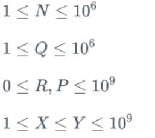# Postman | Software Engineer, Intern | Internship Test Question

You want to buy a laptop. Each laptop has two parameters: Rating & Price. Your task is to buy a laptop with the highest rating within a given price range. Given Q tasks, each query consisting of price range required, you have to print the highest rated laptop that can be bought within that price range.

Input format:

• The first line contains N denoting the number of inputs.
• The following N lines contain P & R denoting price and range of a laptop.
• Next line contains Q denoting the number of queries.
• The following Q lines contain two integers X & Y denoting the price range (inclusive).

Output format:
For each task Q, print the highest rating that can be bought within the range.
If you cannot get any laptop within the range, print -1.

Constraints:Sample Input:
5
1000 300
1100 400
1300 200
1700 500
2000 600
3
1000 1400
1700 1900
0 2000

Sample Output:
400
500
600

My approach:

1. Create a rating array and initialize all its elements with -1.
2. For every price, fill the array with the maximum rating possible till that price.
3. Continue till the maximum price/last element of rating array.
4. Return rating[Y], because Y > X always.

My problem:
The above approach requires huge memory (atleast in C++) as the rating array will contain 10^9 elements (because price range is from 1 to 10^9). This is not possible because of memory constraints. We can probably use maps/dictionaries here, but then, they are heavy too. This is where I need some guidance.

My solution (C++) (SIGSEGV): But works fine with sample test cases. PS: I know this is the right solution, but array size is the problem here.

``````// This was already given
#define  MAXSIZE 1000001

int solve()
{
//write code here
int rating[MAXSIZE];
fill(rating, rating+MAXSIZE, -1);
for (int i=0; i<N; i++)
rating[P[i]] = R[i];

int maxele = rating;
for (int i=0; i<MAXSIZE; i++)
{
rating[i] = max(maxele, rating[i]);
maxele = max(maxele, rating[i]);
}
return rating[Y];
}
``````

My solution (Py3) (Using dict): Partially accepted (1/5 test cases - 20/100)

``````def Solve(N,Price,Rating,Q,Range):
# return a list of answers of Q queries
ans = {}
returnli = []
for i in range(len(Price)):
ans[Price[i]] = Rating[i]
for i in Range:
a = i
b = i
maxel = -1
for i in range(a, b+1):
if i in ans.keys():
maxel = max(maxel, ans[i])
if (i > max(ans.keys())):
break
returnli.append(maxel)
return returnli
``````
2 Likes

Never do anything in cp which is >=10^8.
Here is the solution:-
1)Sort all the pairs according to their prices.
2)Now, whenever you see a price range, binary search and find the indices of that exact range.
Say,
Price Rating
5 1
2 12
23 34
1 0

After sorting according to the prices:–>

Price Rating
1 (1) 0
2 (2) 12
5 (3) 1
23 (4) 34

Now, if query is:—>(2,25), binary search will give you 2 values:–> (2,4)… It means u have to search maximum value in rating array from indice (2) to indice (4), the ratings from indice 2 to 4 are:—>12,1,34… Answer is max(34).
How to find the maximum number in a range in log(N)…use segment tree!
You are doneI hope explaination given by me clears everything!!Thanks for this! I’ll try to implement the code based on what you suggested. Will post it soon. Thanks again man!